# Team:Rice/Modeling

Introduction

Violacein is a fluorescent reporter with anticancer activity (Carvalho et al., 2006) that has been used in several other iGEM projects (Cambridge 2009, Slovenia 2010, Johns Hopkins 2011, UCSF 2012). Although it would be a good pigment candidate for our project, it has a complex synthetic pathway requiring five specialized enzymes and oxygen (Fig. 2). It also presents multiple off-path reactions that can reduce the efficiency of the pathway. Before building constructs to use for violacein production, we needed to find a way to determine which promoters to use for the five genes involved in the pathway. Although there are studies focused on the optimization of the production of violacein (Lee et al., 2013), none of the studies give a biochemical model of the rates of the reactions that take place in the bacteria.

Objective

Create a biochemical model of the violacein production based on the synthetic pathway and violacein production data from bacteria with different promoters for each of the five genes involved in the pathway.

Model Assumptions

1. The rate of dilution of the enzymes and the intermediaries is much greater than its degradation (for example by ubiquitination for the proteins or by conversion to products not included on the pathway)
2. There is no saturation of the enzymes and all the reactions will follow the law of mass action
3. Independence of external factors such as oxygen and NADH in the reactions
4. None of the reactions are reversible
We use the mass action kinetics because this type of equation only requires one parameter for reaction and is less susceptible to overdosing

Model Building Process

1. Modeling Promoter Strength

Because a major goal of the model is to predict the effects of the selection of promoters on the final production of violacein, we decided to find a way to characterize promoters first. To simplify the computation, we used the promoter strength as a single standard to characterize the promoters. Moreover, we assumed the degradation rate of proteins only depends on the growth rate of E.coli. Then, every enzyme has the same degradation rate. The bacteriophage T7 promoter has been widely used for protein expression and purification (Jones et al., 2013), so we used data of five mutant T7 promoters to create a proof-of-concept model. If this model was functional, we could implement the same modeling technique to the promoters we were working with.The five mutant T7 promoters have distinct promoter strength over time after induction. The experimental data from the literature are shown in the figure below (Jones et al., 2013).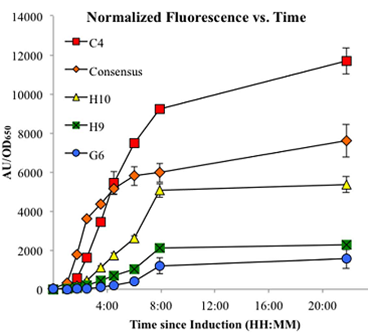The first step of our model is to describe the rate of change of enzymes based on promoter strength. Here we assumed that the enzyme production rate is directly proportional to strength of the promoter. Therefore, we were able to use a mass-action kinetics equation of promoters to describe the enzyme concentration. The equation is shown below: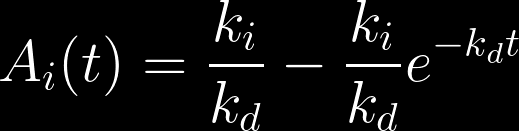In this equation, Ai is the concentration of enzyme i, ki­ is the production rate of each enzyme i, kd is the degradation rate of all enzymes, and t is time. By solving this equation, we derived the equation of enzyme concentration against time.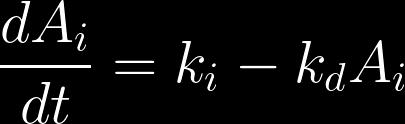Since we assumed that the promoter strength is proportional to the promoter concentration, we would use the equation to fit our data using least squares method (Fig. 1).Figure 1. Linear regressions fitted to normalized fluorescence vs time. The circles represent data from Jones et al., 2013. The solid lines are our regression lines. The colors indicate with which promoters the circles and lines correspond.

In general, the regression lines are able to capture the change of strength of each enzyme over time. In this way, the parameters are determined. The table below lists the parameter values.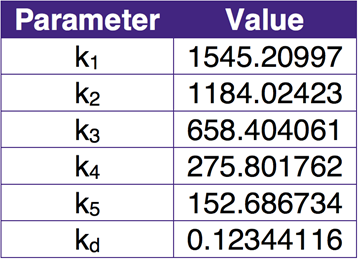Table 1. Parameters realted to promoter strength and degradation of molecules. In the table, ki­ (i = 1,2,3,4,5) are the production rate coefficients of promoter I (i = 1,2,3,4,5), and kd is the degradation rate coefficient of all promoters.

2. Modeling the Steady-state Violacein Yield

After we finished the regression model of each promoter, we created a second model to describe the violacein biosynthetic pathway. The pathway (Fig. 2) involves five enzyme-catalyzed reactions and one non-enzymatic reaction (Lee et al, 2013).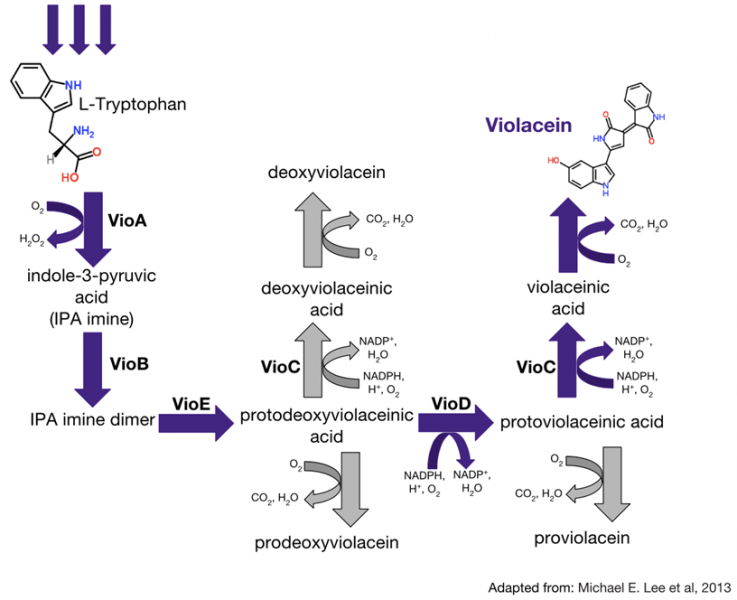Figure 2. Violacein synthetic pathway. The purple arrows highlight the five enzymatic and one non-enzymatic steps of violacein production from two molecules of tryptophan. The five enzymes are indicated by bolding (VioA, VioB, etc.).

The model was developed as three major parts. A pseudocode of this model is provided here.
Define ODE System
1. Calculate the production and degradation rate of each molecule in the pathway from the concentration of reagents and parameters.
2. Obtain the rate of change of each molecule based on the production and degradation rates.
Solve the System of Nonlinear Equations at Steady State
1. Solve the system of nonlinear equations at steady state starting at an initial guess X0.
2. Use the result as a new initial guess; repeat the numerical method to solve the system of equations again.
3. Calculate the relative error of each chemical in the new result.
4. If the maximum error is smaller than 0.0001%, output violacein concentration at steady state as the final result.
Optimize Parameters to Fit Experimental Data
1. Set the initial guess of the parameters.
2. Load the data from literature, which include the choice of promoter for each gene and the corresponding violacein yield determined experimentally.
3. For each promoter selection scenario, pass the promoter types and the temporary parameters to the steady-state model.
4. Obtain the violacein yield predicted by the steady-state model for each promoter selection scenario.
5. Compute the residual sum of squares (RSS) of between the predicted violacein yields and the violacein yields given by experiment.
6. Determine the optimal parameters by minimizing the RSS (least square method).

Using the principles of mass action kinetics, we derived the system of ODE equations in the model. The equations involves 17 parameters (Table 2). Five parameters (kA, kB, kC, kD and kE) are related to the production rates of the five enzymes, which depend only the strength of the promoter type. Another parameter, kd, is the degradation coefficient of all molecules due to the growth of E.coli. The value of this parameter is fixed and shown in Table1. In addition to these known parameters, the equations include 11 undetermined parameters related to the reaction rates at specific steps in the violacein synthetic pathway. As described in the pseudocode, we used least square regression to determine the optimal values of these parameters.

Each one of the11 differential equations describes the rate of change of specific molecule in the system. The equations consider the production, consumption, and degradation rates of the molecules. Degradation of molecules is described by first order decay. Therefore, the rate of degradation of a molecule depends on a degradation constant and the degradation coefficient. The degradation coefficient is identical for all molecules since it only depends on E.coli growth rate.

Differential Equations in the Model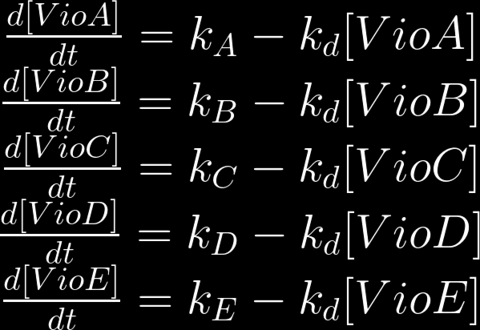Results

Our model is able to compute the average violacein yields for all the strains tested experimentally, but can not capture the difference of violacein yield with different promoters strengths. The comparison between the violacein yields determined by experiments and those predicted by our model is shown in Figure 3. The optimal parameters determined by the model are listed in Table 2.Figure 3. VIolacein yield with different promoter combinations. This graph compares the violacein found for various promoter combinations determined by Jones et al., 2013 (shown in blue) with the violacein concentrations that our model predicted for the same promoter combinations. The root-mean-square error (RMSE) is 52.04.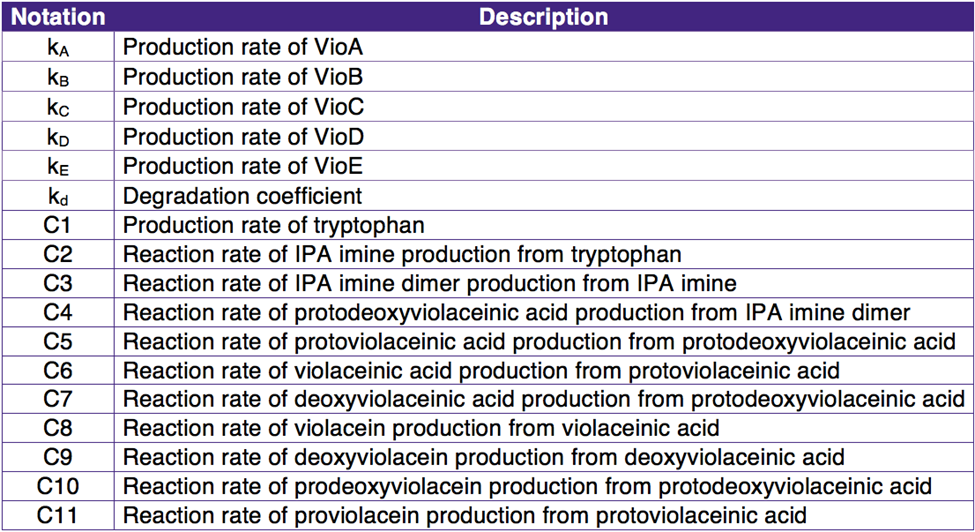Table 2. Notations of parameters.

Discussion

The current model is not able to show the expected dependence of violacein yield on promoter strength. After reevaluating our assumptions, we identified some potential flaws of the model that might cause the unexpected results.

One of the assumptions from our model is that the rate of production of L-tryptophan is constant and independent of the promoter strength. Jones el al. suggest that the L-tryptophan production rate may be affected by the metabolic burden of the production of the recombinant enzymes (VioA, VioB, etc.). This phenomenon may be caused by the depletion of essential metabolic resource, such as amino acids, mRNA and ATP. Therefore, the L-tryptophan production rate might need to be dependent on enzymes production rates.

Another effect that we didn’t consider is the saturation of the enzymes. To improve our model, we could include these effects by employing Michaelis-Menten Kinetics equations in our next step. Nevertheless, we have been cautious about including this in our model, since increasing the number of parameters, without increasing the number of data points usually causes the overfitting of the model.

Finally, since the violacein pathway has not been fully characterized, it is possible that we ignored some reactions in the complete pathway. Moreover, there may be feedback loops that regulate the pathway. We will need to investigate these possible components and incorporate them into our model if they prove to be present in the pathway.

Conclusion

Here we present a method to fit a model of violacein production in E.coli to experimental data of violacein yield with different promoters using nonlinear regression. Although it fails to calculate the dependence on promoter strength, our model is able predict the average violacein concentration. We expect that small changes on the model, such as including a L-tryptophan production dependence of the metabolic burden, would allow us to successfully predict the violacein production in response to the variation of promoter strength. Once the predictive model is complete, we will be able to find the strains that lead to optimal violacein yield computationally.
References
1. Carvalho, D. D., Costa, F. T. M., Duran, N., & Haun, M. (2006). Cytotoxic activity of violacein in human colon cancer cells. Toxicology in Vitro, 20(8), 1514–1521.
http://dx.doi.org/10.1016/j.tiv.2006.06.007
2. Jones, J. A., Vernacchio, V. R., Lachance, D. M., Lebovich, M., Fu, L., Shirke, A. N., … Koffas, M. A. G. (2015). ePathOptimize: A Combinatorial Approach for Transcriptional Balancing of Metabolic Pathways. Scientific Reports, 5, 11301.
http://doi.org/10.1038/srep11301
3. Lee, M. E., Aswani, A., Han, A. S., Tomlin, C. J., & Dueber, J. E. (2013). Expression-level optimization of a multi-enzyme pathway in the absence of a high-throughput assay. Nucleic Acids Research, 41(22), 10668–10678.
http://doi.org/10.1093/nar/gkt809